C++虚函数表解析

年薪达不到25W大数据工程师、拿不到Offer全额退款->>>C++中的虚函数的作用主要是实现了多态的机制。关于多态，简而言之就是用父类型别的指针指向其子类的实例，然后通过父类的指针调用实际子类的成员函数。这种技术可以让父类的指针有“多种形态”，这是一种泛型技术。所谓泛型技术，说白了就是试图使用不变的代码来实现可变的算法。比如：模板技术，RTTI技术，虚函数技术，要么是试图做到在编译时决议，要么试图做到运行时决议。

虚函数表

class Base {

public:

virtual void f() { cout << "Base::f" << endl; }

virtual void g() { cout << "Base::g" << endl; }

virtual void h() { cout << "Base::h" << endl; }

};

typedef void(*Fun)(void);

Base b;

Fun pFun = NULL;

cout << "虚函数表地址：" << (int*)(&b) << endl;

cout << "虚函数表 — 第一个函数地址：" << (int*)*(int*)(&b) << endl;

// Invoke the first virtual function

pFun = (Fun)*((int*)*(int*)(&b));

pFun();

Base::f

(Fun)*((int*)*(int*)(&b)+0); // Base::f()

(Fun)*((int*)*(int*)(&b)+1); // Base::g()

(Fun)*((int*)*(int*)(&b)+2); // Base::h()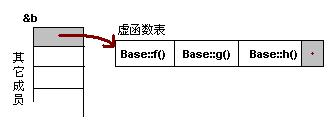一般继承（无虚函数覆盖）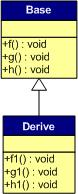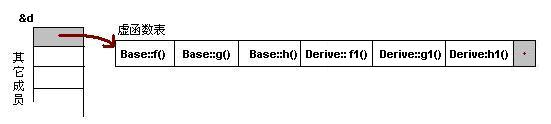1）虚函数按照其声明顺序放于表中。

2）父类的虚函数在子类的虚函数前面。

一般继承（有虚函数覆盖）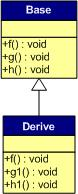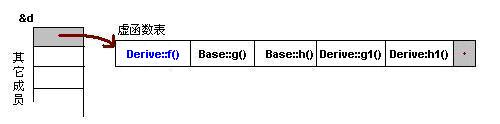1）覆盖的f()函数被放到了虚表中原来父类虚函数的位置。

2）没有被覆盖的函数依旧。

Base *b = new Derive();

b->f();

多重继承（无虚函数覆盖）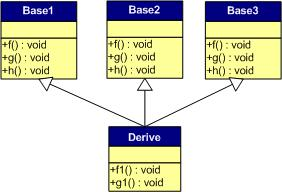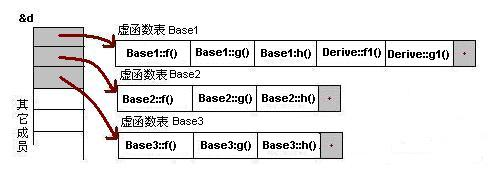1） 每个父类都有自己的虚表。

2） 子类的成员函数被放到了第一个父类的表中。（所谓的第一个父类是按照声明顺序来判断的）

多重继承（有虚函数覆盖）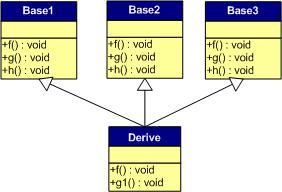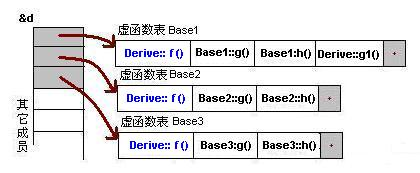Derive d;

Base1 *b1 = &d;

Base2 *b2 = &d;

Base3 *b3 = &d;

b1->f(); //Derive::f()

b2->f(); //Derive::f()

b3->f(); //Derive::f()

b1->g(); //Base1::g()

b2->g(); //Base2::g()

b3->g(); //Base3::g()

安全性

Base1 *b1 = new Derive();

b1->f1(); //编译出错

class Base {

private:

virtual void f() { cout << "Base::f" << endl; }

};

class Derive : public Base{

};

typedef void(*Fun)(void);

void main() {

Derive d;

Fun pFun = (Fun)*((int*)*(int*)(&d)+0);

pFun();

}

结束语

C++这门语言是一门Magic的语言，对于程序员来说，我们似乎永远摸不清楚这门语言背着我们在干了什么。需要熟悉这门语言，我们就必需要了解C++里面的那些东西，需要去了解C++中那些危险的东西。不然，这是一种搬起石头砸自己脚的编程语言